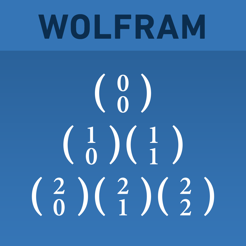## Capturas de pantalla

•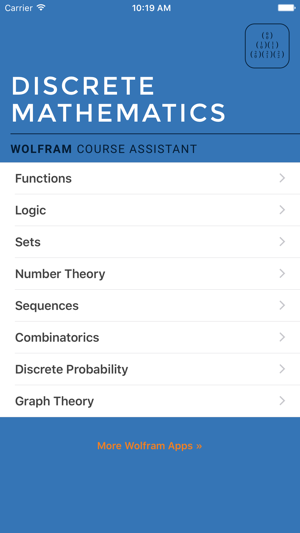•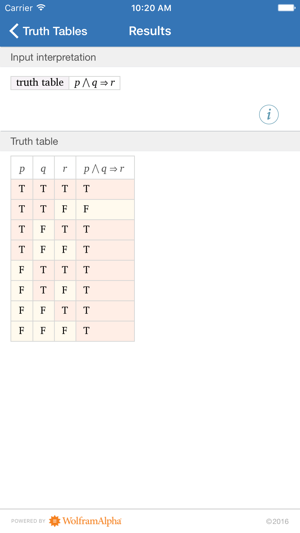•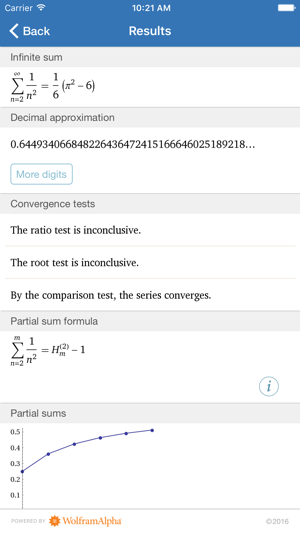•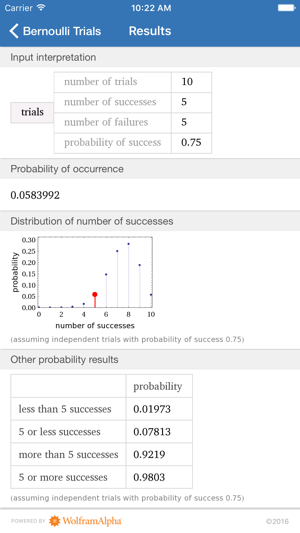•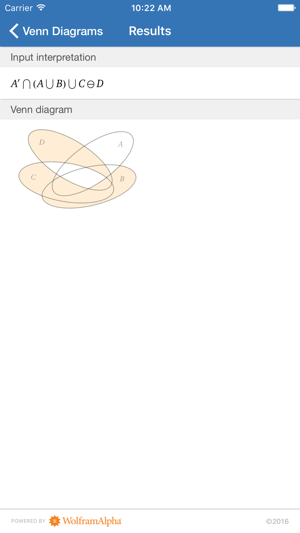## Descripción

Taking discrete mathematics? Then you need the Wolfram Discrete Mathematics Course Assistant. This app for discrete math—from the world leader in math software—will help you work through your homework problems, ace your tests, and learn discrete math concepts. The Discrete Mathematics Course Assistant solves your specific discrete math problems on the fly, providing answers to a broad range of subjects.

- Do function calculations like domain and range, image and preimage, and inverse and growth
- Compute logic problems like minimal forms, implications, propositions, and bitwise operations
- Calculate set functions like power set, basic set operations, complement, and Venn diagrams
- Use the Number Theory section for division, modular arithmetic, prime numbers, special numbers, and integer functions
- Do sequence computations like summation, product, and limit of a sequence
- Compute permutation and combinatorics questions, including derangements and permutations of list or finite relations and Pascal's triangle
- Use the discrete probability section for Bernoulli trial equations and view statistics on coin and dice probabilities or view various distribution given the probability of success
- View information on basic, named, or custom graphs in our Graph Theory section

The Wolfram Discrete Mathematics Course Assistant is powered by the Wolfram|Alpha computational knowledge engine and is created by Wolfram Research, makers of Mathematica—the world's leading software system for mathematical research and education.

The Wolfram Discrete Mathematics Course Assistant draws on the computational power of Wolfram|Alpha's supercomputers over a 3G, 4G, or Wi-Fi connection.

Versión 1.3.1

- Bug fixes

3.0 de 5
1 valoración

1 valoración

## Información

Vendedor
Wolfram Group LLC
Tamaño
14.6 MB
Categoría
Educación

Requiere iOS 9.3 o posterior. Compatible con iPhone, iPad y iPod touch.

Idiomas

Inglés

4+
Precio
\$99.00

•